# R S Aggarwal Solutions for Class 10 Maths Exercise 19B Chapter 19 Probability

R S Aggarwal Solutions for Class 10 helpful for best score in board and competitive exams. Chapter 19 exercise 19b study material is now available online and in the PDF format at BYJU’S. Students are advised to study from R S Aggarwal solution to score good marks in the class 10 examination. Download pdf of Class 10 Chapter 19 Maths R S Aggarwal Solutions and practice to score good marks.

## Download PDF of R S Aggarwal Solutions for Class 10 Maths Chapter 19 Probability Exercise 19B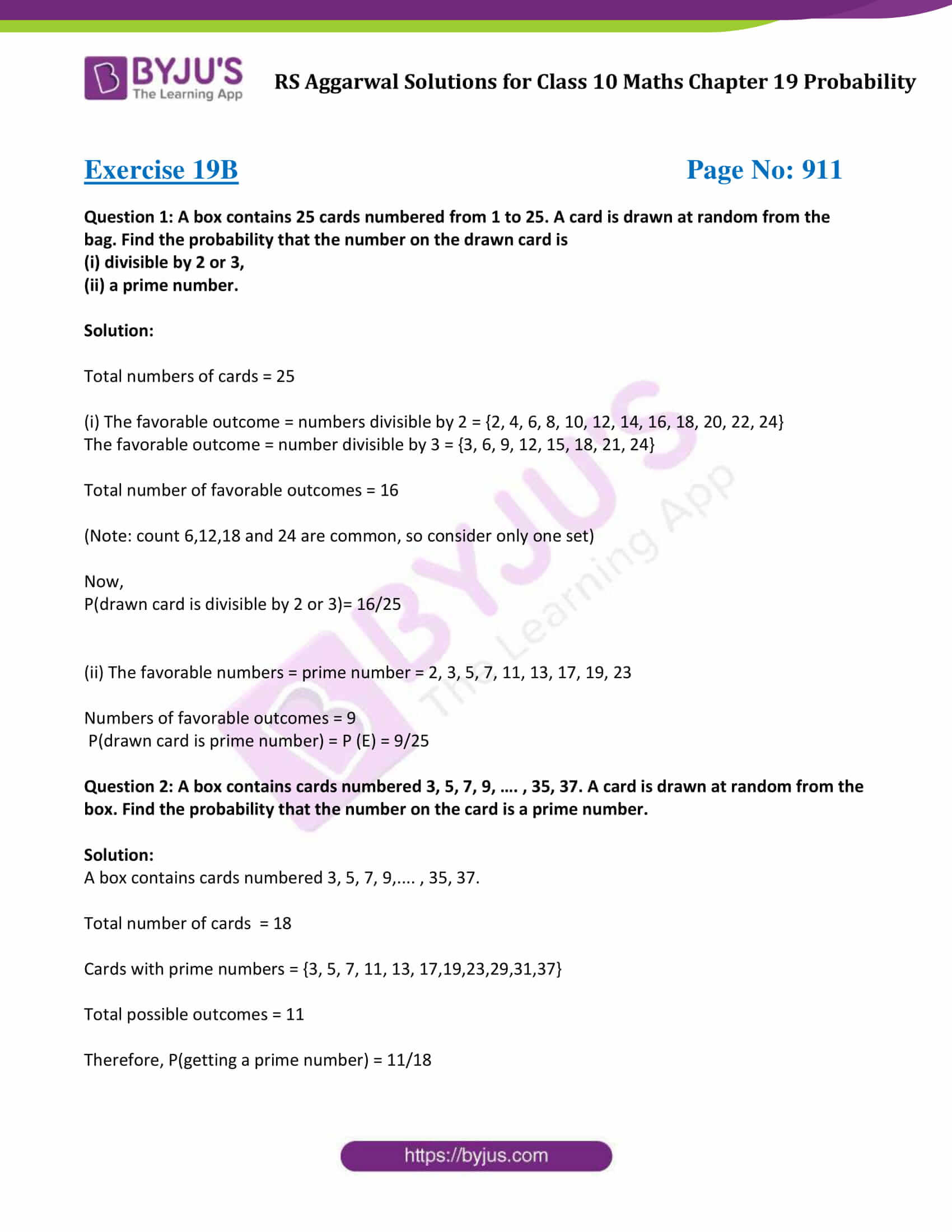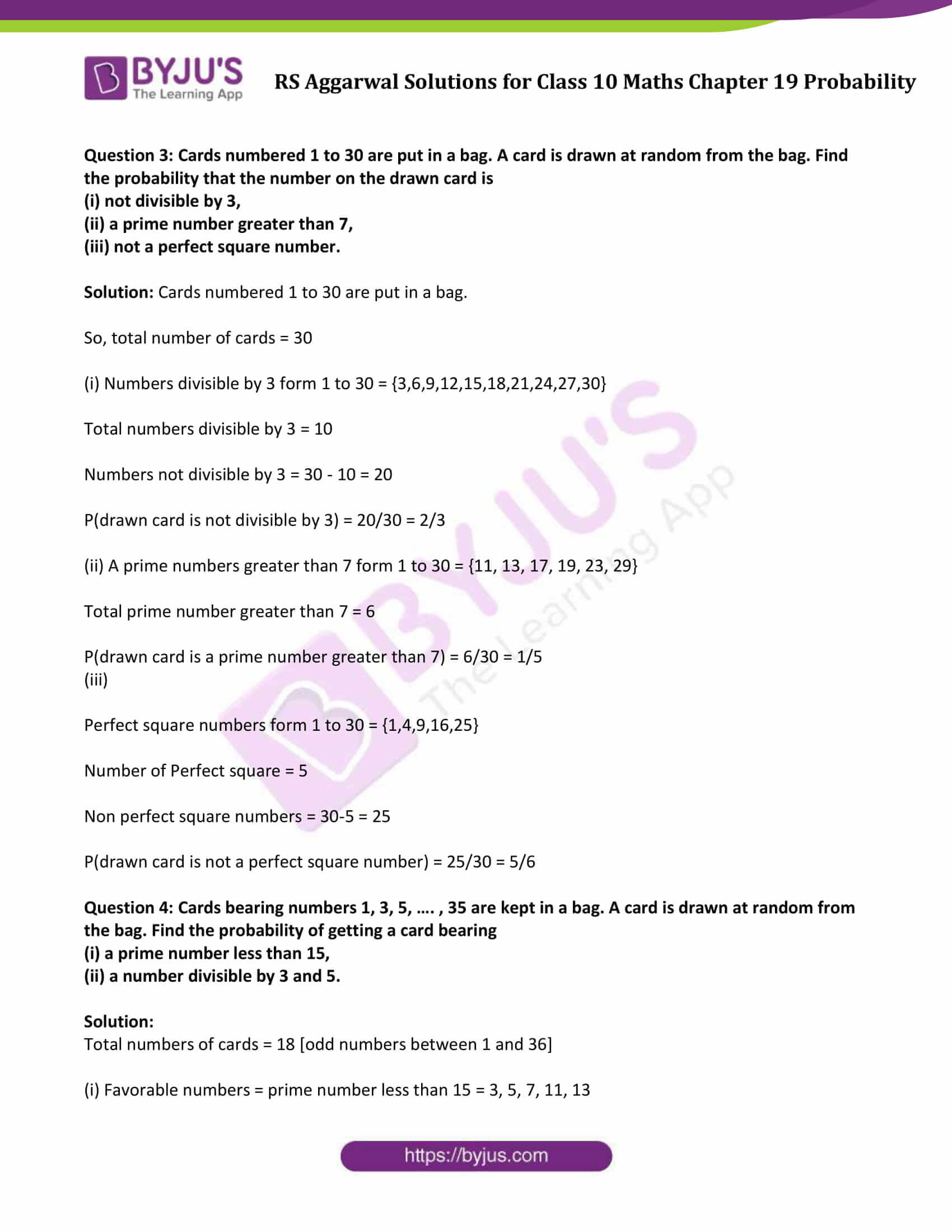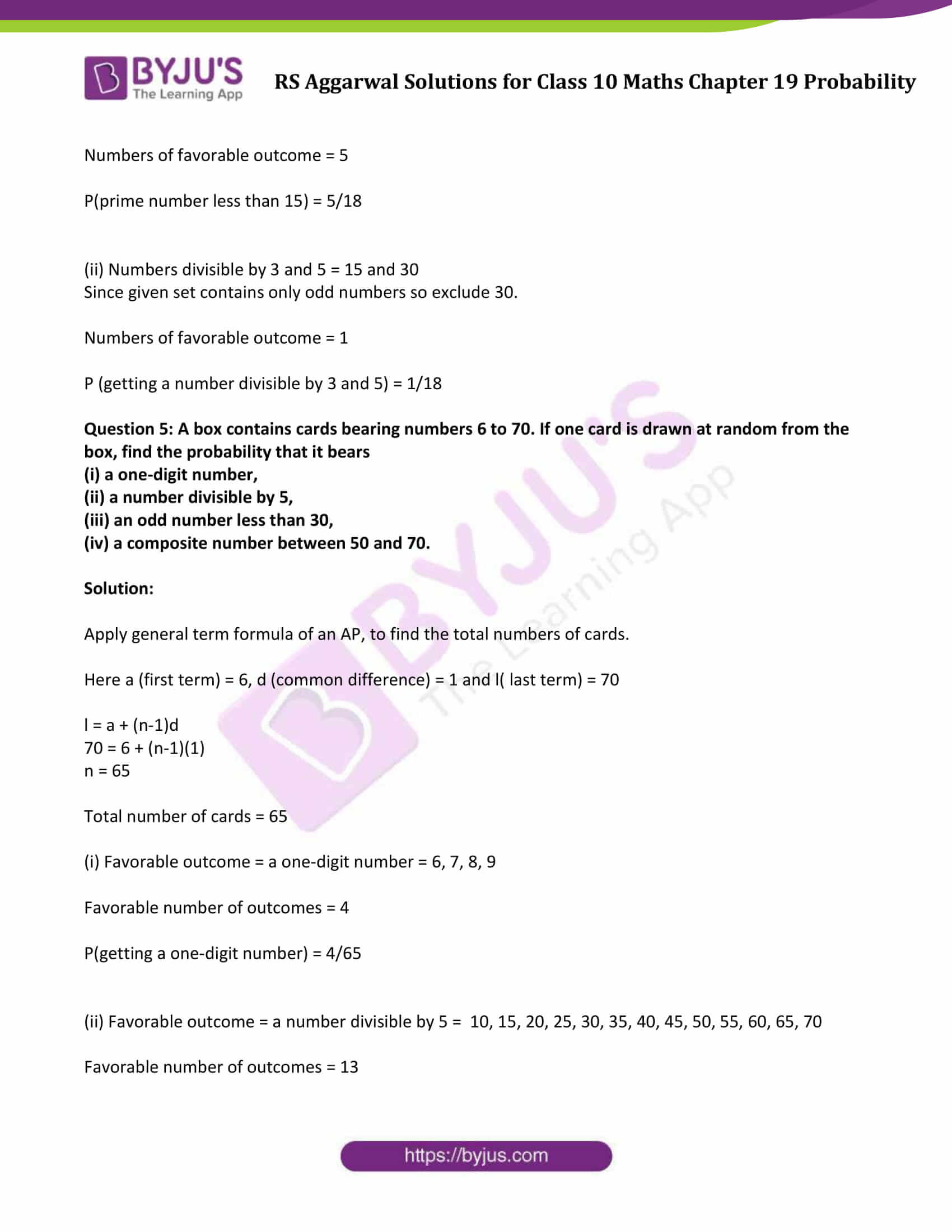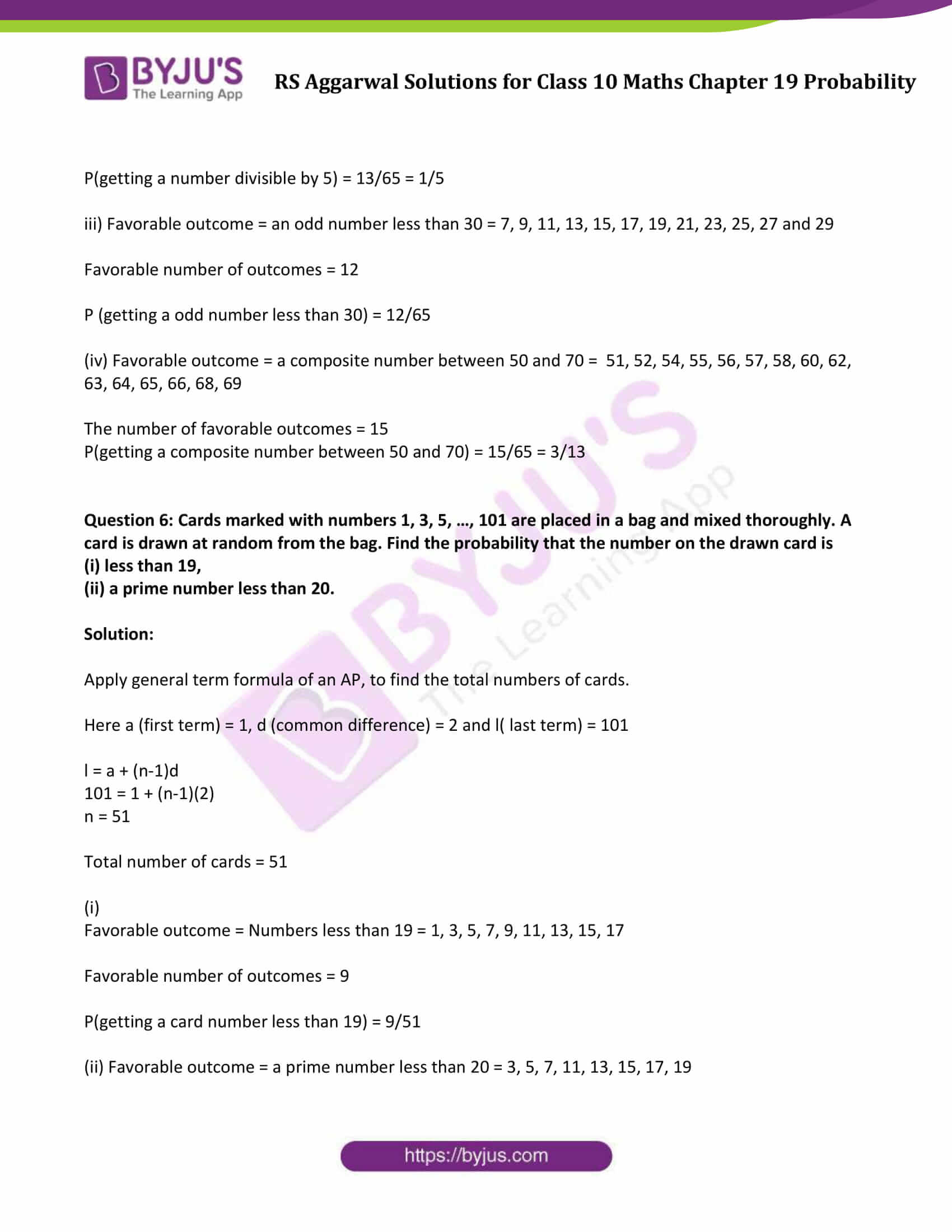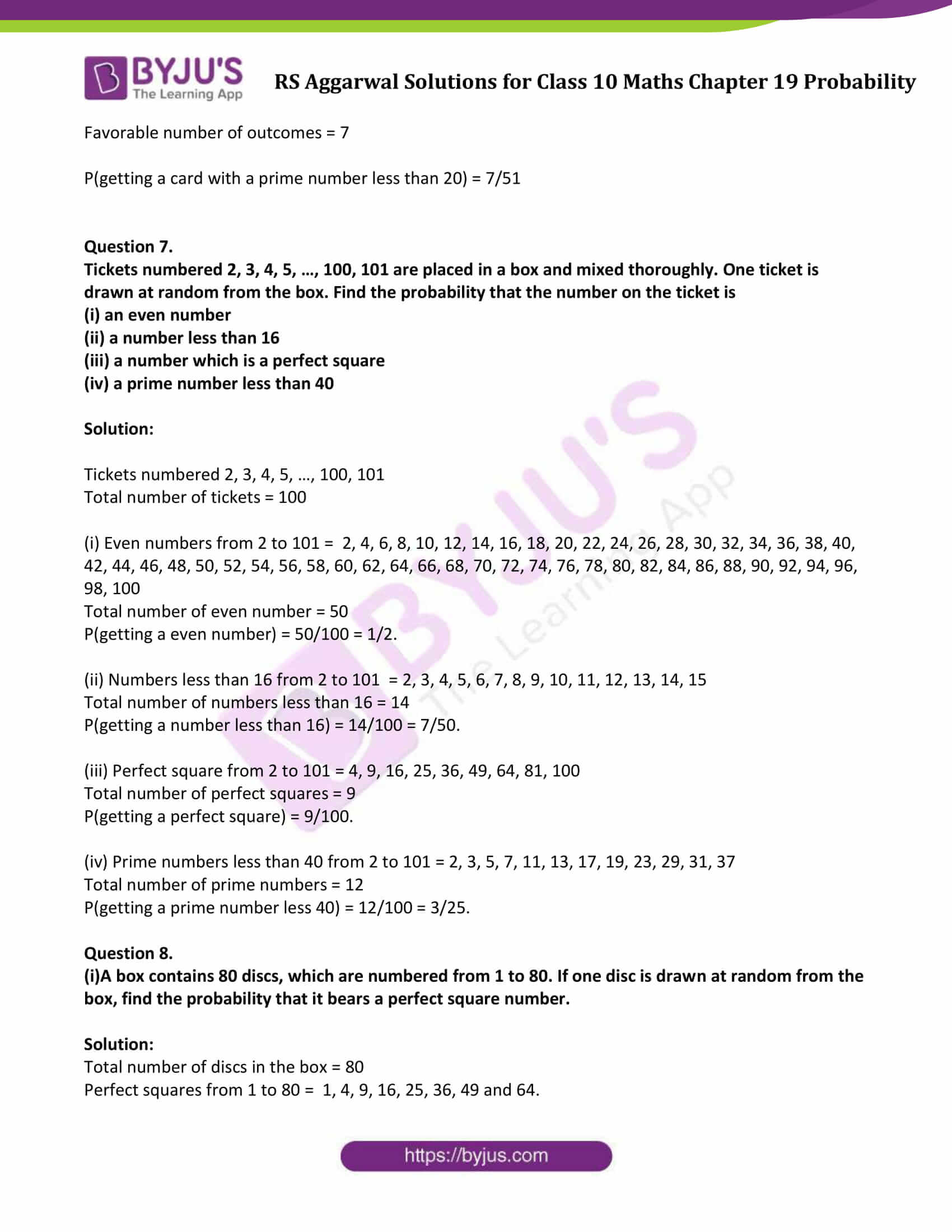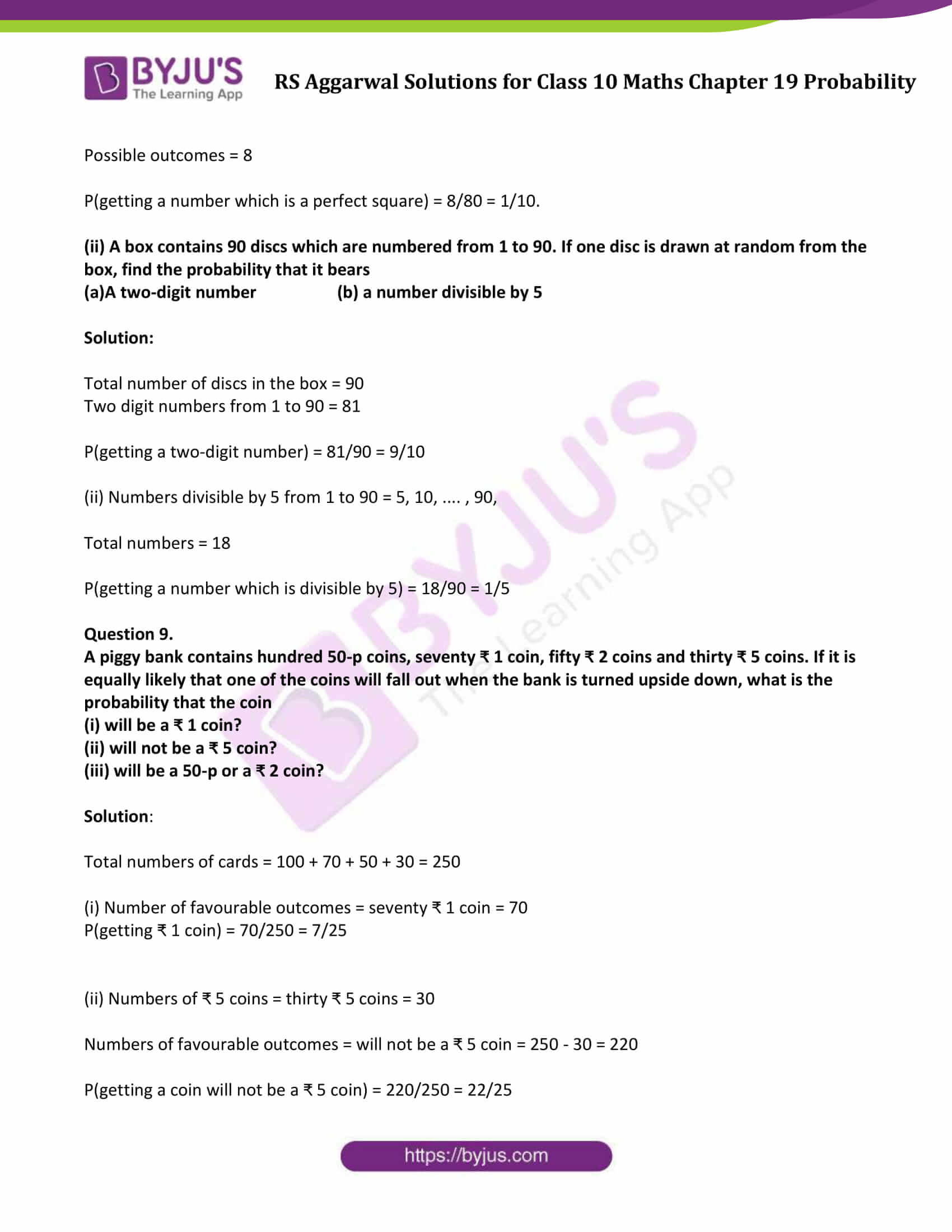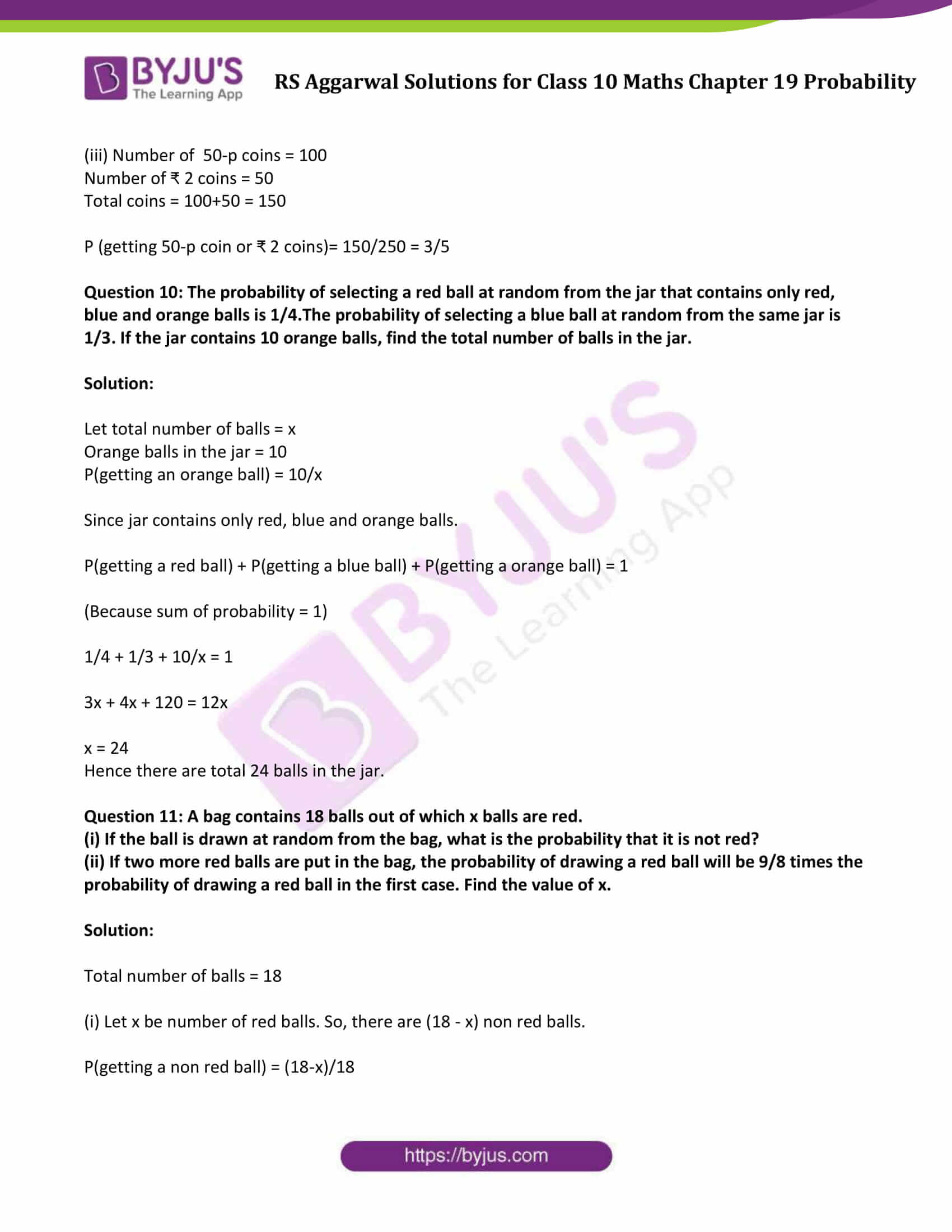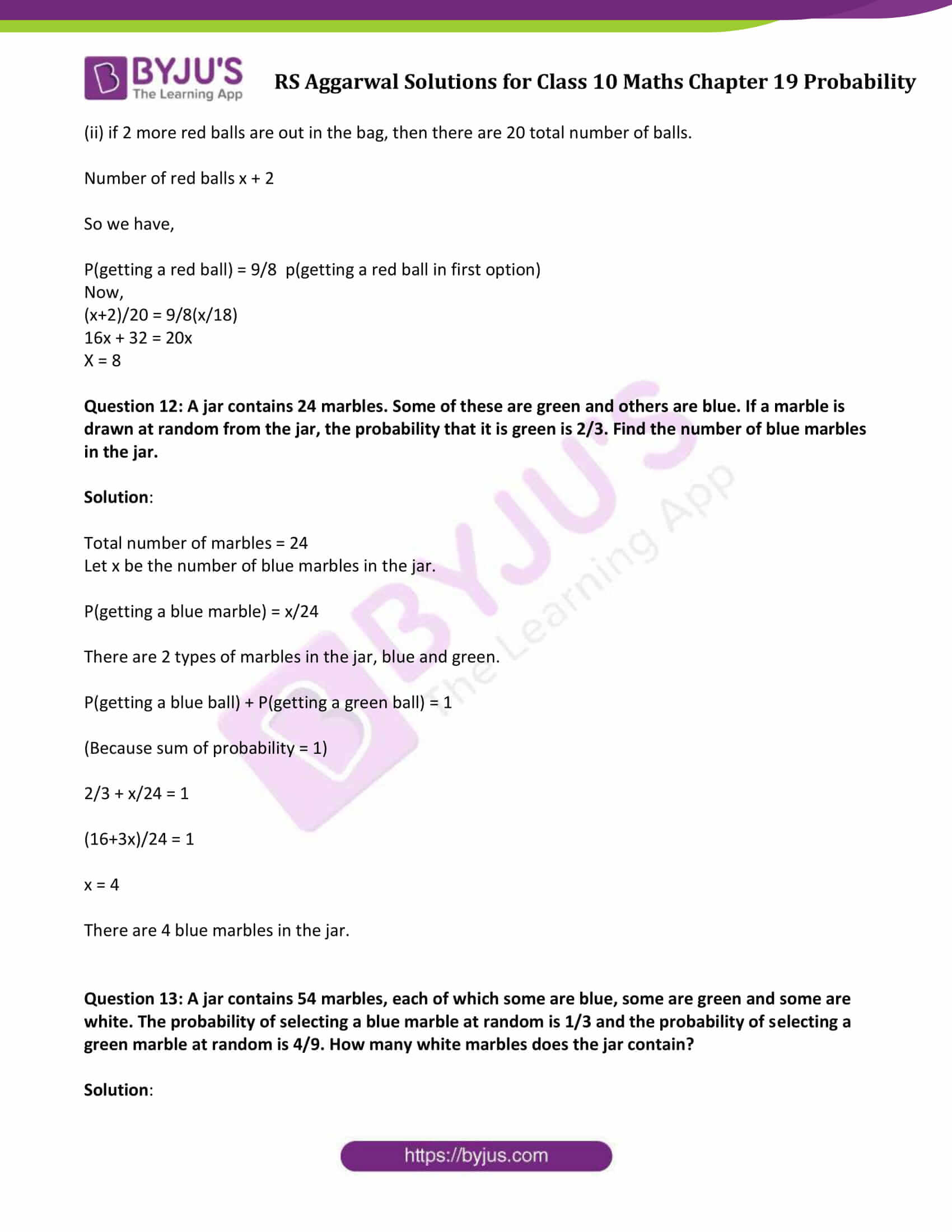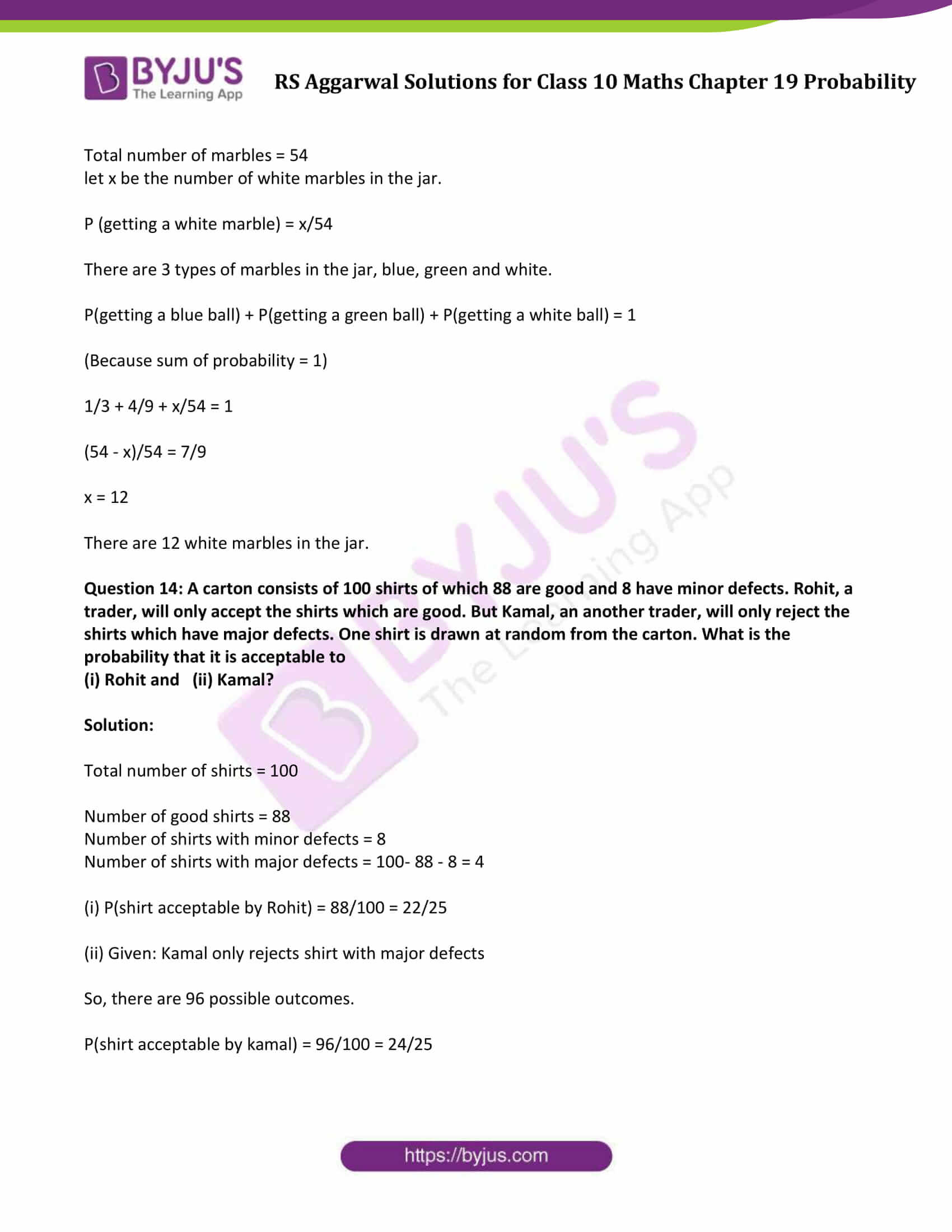## Access other exercise solutions of Class 10 Maths Chapter 19 Probability

Exercise 19A Solutions

### Access Solutions to Maths R S Aggarwal Class 10 Chapter 19 Probability Exercise 19B

Question 1: A box contains 25 cards numbered from 1 to 25. A card is drawn at random from the bag. Find the probability that the number on the drawn card is

(i) divisible by 2 or 3,

(ii) a prime number.

Solution:

Total numbers of cards = 25

(i) The favorable outcome = numbers divisible by 2 = {2, 4, 6, 8, 10, 12, 14, 16, 18, 20, 22, 24}

The favorable outcome = number divisible by 3 = {3, 6, 9, 12, 15, 18, 21, 24}

Total number of favorable outcomes = 16

(Note: count 6,12,18 and 24 are common, so consider only one set)

Now,

P(drawn card is divisible by 2 or 3)= 16/25

(ii) The favorable numbers = prime number = 2, 3, 5, 7, 11, 13, 17, 19, 23

Numbers of favorable outcomes = 9

P(drawn card is prime number) = P (E) = 9/25

Question 2: A box contains cards numbered 3, 5, 7, 9, …. , 35, 37. A card is drawn at random from the box. Find the probability that the number on the card is a prime number.

Solution:

A box contains cards numbered 3, 5, 7, 9,…. , 35, 37.

Total number of cards = 18

Cards with prime numbers = {3, 5, 7, 11, 13, 17,19,23,29,31,37}

Total possible outcomes = 11

Therefore, P(getting a prime number) = 11/18

Question 3: Cards numbered 1 to 30 are put in a bag. A card is drawn at random from the bag. Find the probability that the number on the drawn card is

(i) not divisible by 3,

(ii) a prime number greater than 7,

(iii) not a perfect square number.

Solution: Cards numbered 1 to 30 are put in a bag.

So, total number of cards = 30

(i) Numbers divisible by 3 form 1 to 30 = {3,6,9,12,15,18,21,24,27,30}

Total numbers divisible by 3 = 10

Numbers not divisible by 3 = 30 – 10 = 20

P(drawn card is not divisible by 3) = 20/30 = 2/3

(ii) A prime numbers greater than 7 form 1 to 30 = {11, 13, 17, 19, 23, 29}

Total prime number greater than 7 = 6

P(drawn card is a prime number greater than 7) = 6/30 = 1/5

(iii)

Perfect square numbers form 1 to 30 = {1,4,9,16,25}

Number of Perfect square = 5

Non perfect square numbers = 30-5 = 25

P(drawn card is not a perfect square number) = 25/30 = 5/6

Question 4: Cards bearing numbers 1, 3, 5, …. , 35 are kept in a bag. A card is drawn at random from the bag. Find the probability of getting a card bearing

(i) a prime number less than 15,

(ii) a number divisible by 3 and 5.

Solution:

Total numbers of cards = 18 [odd numbers between 1 and 36]

(i) Favorable numbers = prime number less than 15 = 3, 5, 7, 11, 13

Numbers of favorable outcome = 5

P(prime number less than 15) = 5/18

(ii) Numbers divisible by 3 and 5 = 15 and 30

Since given set contains only odd numbers so exclude 30.

Numbers of favorable outcome = 1

P (getting a number divisible by 3 and 5) = 1/18

Question 5: A box contains cards bearing numbers 6 to 70. If one card is drawn at random from the box, find the probability that it bears

(i) a one-digit number,

(ii) a number divisible by 5,

(iii) an odd number less than 30,

(iv) a composite number between 50 and 70.

Solution:

Apply general term formula of an AP, to find the total numbers of cards.

Here a (first term) = 6, d (common difference) = 1 and l( last term) = 70

l = a + (n-1)d

70 = 6 + (n-1)(1)

n = 65

Total number of cards = 65

(i) Favorable outcome = a one-digit number = 6, 7, 8, 9

Favorable number of outcomes = 4

P(getting a one-digit number) = 4/65

(ii) Favorable outcome = a number divisible by 5 = 10, 15, 20, 25, 30, 35, 40, 45, 50, 55, 60, 65, 70

Favorable number of outcomes = 13

P(getting a number divisible by 5) = 13/65 = 1/5

iii) Favorable outcome = an odd number less than 30 = 7, 9, 11, 13, 15, 17, 19, 21, 23, 25, 27 and 29

Favorable number of outcomes = 12

P (getting a odd number less than 30) = 12/65

(iv) Favorable outcome = a composite number between 50 and 70 = 51, 52, 54, 55, 56, 57, 58, 60, 62, 63, 64, 65, 66, 68, 69

The number of favorable outcomes = 15

P(getting a composite number between 50 and 70) = 15/65 = 3/13

Question 6: Cards marked with numbers 1, 3, 5, …, 101 are placed in a bag and mixed thoroughly. A card is drawn at random from the bag. Find the probability that the number on the drawn card is

(i) less than 19,

(ii) a prime number less than 20.

Solution:

Apply general term formula of an AP, to find the total numbers of cards.

Here a (first term) = 1, d (common difference) = 2 and l( last term) = 101

l = a + (n-1)d

101 = 1 + (n-1)(2)

n = 51

Total number of cards = 51

(i)

Favorable outcome = Numbers less than 19 = 1, 3, 5, 7, 9, 11, 13, 15, 17

Favorable number of outcomes = 9

P(getting a card number less than 19) = 9/51

(ii) Favorable outcome = a prime number less than 20 = 3, 5, 7, 11, 13, 15, 17, 19

Favorable number of outcomes = 7

P(getting a card with a prime number less than 20) = 7/51

Question 7.

Tickets numbered 2, 3, 4, 5, …, 100, 101 are placed in a box and mixed thoroughly. One ticket is drawn at random from the box. Find the probability that the number on the ticket is

(i) an even number

(ii) a number less than 16

(iii) a number which is a perfect square

(iv) a prime number less than 40

Solution:

Tickets numbered 2, 3, 4, 5, …, 100, 101

Total number of tickets = 100

(i) Even numbers from 2 to 101 = 2, 4, 6, 8, 10, 12, 14, 16, 18, 20, 22, 24, 26, 28, 30, 32, 34, 36, 38, 40, 42, 44, 46, 48, 50, 52, 54, 56, 58, 60, 62, 64, 66, 68, 70, 72, 74, 76, 78, 80, 82, 84, 86, 88, 90, 92, 94, 96, 98, 100

Total number of even number = 50

P(getting a even number) = 50/100 = 1/2.

(ii) Numbers less than 16 from 2 to 101 = 2, 3, 4, 5, 6, 7, 8, 9, 10, 11, 12, 13, 14, 15

Total number of numbers less than 16 = 14

P(getting a number less than 16) = 14/100 = 7/50.

(iii) Perfect square from 2 to 101 = 4, 9, 16, 25, 36, 49, 64, 81, 100

Total number of perfect squares = 9

P(getting a perfect square) = 9/100.

(iv) Prime numbers less than 40 from 2 to 101 = 2, 3, 5, 7, 11, 13, 17, 19, 23, 29, 31, 37

Total number of prime numbers = 12

P(getting a prime number less 40) = 12/100 = 3/25.

Question 8.

(i)A box contains 80 discs, which are numbered from 1 to 80. If one disc is drawn at random from the box, find the probability that it bears a perfect square number.

Solution:

Total number of discs in the box = 80

Perfect squares from 1 to 80 = 1, 4, 9, 16, 25, 36, 49 and 64.

Possible outcomes = 8

P(getting a number which is a perfect square) = 8/80 = 1/10.

(ii) A box contains 90 discs which are numbered from 1 to 90. If one disc is drawn at random from the box, find the probability that it bears

(a)A two-digit number (b) a number divisible by 5

Solution:

Total number of discs in the box = 90

Two digit numbers from 1 to 90 = 81

P(getting a two-digit number) = 81/90 = 9/10

(ii) Numbers divisible by 5 from 1 to 90 = 5, 10, …. , 90,

Total numbers = 18

P(getting a number which is divisible by 5) = 18/90 = 1/5

Question 9.

A piggy bank contains hundred 50-p coins, seventy ₹ 1 coin, fifty ₹ 2 coins and thirty ₹ 5 coins. If it is equally likely that one of the coins will fall out when the bank is turned upside down, what is the probability that the coin

(i) will be a ₹ 1 coin?

(ii) will not be a ₹ 5 coin?

(iii) will be a 50-p or a ₹ 2 coin?

Solution:

Total numbers of cards = 100 + 70 + 50 + 30 = 250

(i) Number of favourable outcomes = seventy ₹ 1 coin = 70

P(getting ₹ 1 coin) = 70/250 = 7/25

(ii) Numbers of ₹ 5 coins = thirty ₹ 5 coins = 30

Numbers of favourable outcomes = will not be a ₹ 5 coin = 250 – 30 = 220

P(getting a coin will not be a ₹ 5 coin) = 220/250 = 22/25

(iii) Number of 50-p coins = 100

Number of ₹ 2 coins = 50

Total coins = 100+50 = 150

P (getting 50-p coin or ₹ 2 coins)= 150/250 = 3/5

Question 10: The probability of selecting a red ball at random from the jar that contains only red, blue and orange balls is 1/4.The probability of selecting a blue ball at random from the same jar is 1/3. If the jar contains 10 orange balls, find the total number of balls in the jar.

Solution:

Let total number of balls = x

Orange balls in the jar = 10

P(getting an orange ball) = 10/x

Since jar contains only red, blue and orange balls.

P(getting a red ball) + P(getting a blue ball) + P(getting a orange ball) = 1

(Because sum of probability = 1)

1/4 + 1/3 + 10/x = 1

3x + 4x + 120 = 12x

x = 24

Hence there are total 24 balls in the jar.

Question 11: A bag contains 18 balls out of which x balls are red.

(i) If the ball is drawn at random from the bag, what is the probability that it is not red?

(ii) If two more red balls are put in the bag, the probability of drawing a red ball will be 9/8 times the probability of drawing a red ball in the first case. Find the value of x.

Solution:

Total number of balls = 18

(i) Let x be number of red balls. So, there are (18 – x) non red balls.

P(getting a non red ball) = (18-x)/18

(ii) if 2 more red balls are out in the bag, then there are 20 total number of balls.

Number of red balls x + 2

So we have,

P(getting a red ball) = 9/8 p(getting a red ball in first option)

Now,

(x+2)/20 = 9/8(x/18)

16x + 32 = 20x

X = 8

Question 12: A jar contains 24 marbles. Some of these are green and others are blue. If a marble is drawn at random from the jar, the probability that it is green is 2/3. Find the number of blue marbles in the jar.

Solution:

Total number of marbles = 24

Let x be the number of blue marbles in the jar.

P(getting a blue marble) = x/24

There are 2 types of marbles in the jar, blue and green.

P(getting a blue ball) + P(getting a green ball) = 1

(Because sum of probability = 1)

2/3 + x/24 = 1

(16+3x)/24 = 1

x = 4

There are 4 blue marbles in the jar.

Question 13: A jar contains 54 marbles, each of which some are blue, some are green and some are white. The probability of selecting a blue marble at random is 1/3 and the probability of selecting a green marble at random is 4/9. How many white marbles does the jar contain?

Solution:

Total number of marbles = 54

let x be the number of white marbles in the jar.

P (getting a white marble) = x/54

There are 3 types of marbles in the jar, blue, green and white.

P(getting a blue ball) + P(getting a green ball) + P(getting a white ball) = 1

(Because sum of probability = 1)

1/3 + 4/9 + x/54 = 1

(54 – x)/54 = 7/9

x = 12

There are 12 white marbles in the jar.

Question 14: A carton consists of 100 shirts of which 88 are good and 8 have minor defects. Rohit, a trader, will only accept the shirts which are good. But Kamal, an another trader, will only reject the shirts which have major defects. One shirt is drawn at random from the carton. What is the probability that it is acceptable to

(i) Rohit and (ii) Kamal?

Solution:

Total number of shirts = 100

Number of good shirts = 88

Number of shirts with minor defects = 8

Number of shirts with major defects = 100- 88 – 8 = 4

(i) P(shirt acceptable by Rohit) = 88/100 = 22/25

(ii) Given: Kamal only rejects shirt with major defects

So, there are 96 possible outcomes.

P(shirt acceptable by kamal) = 96/100 = 24/25

## R S Aggarwal Solutions for Class 10 Maths Chapter 19 Probability Exercise 19B

Class 10 Maths Chapter 19 Probability Exercise 19B is based on following topics and subtopics:

• Probability of occurrence of an event
• Possible outcomes in various experiments
• Different types of events
• Word Problems in real-life situations